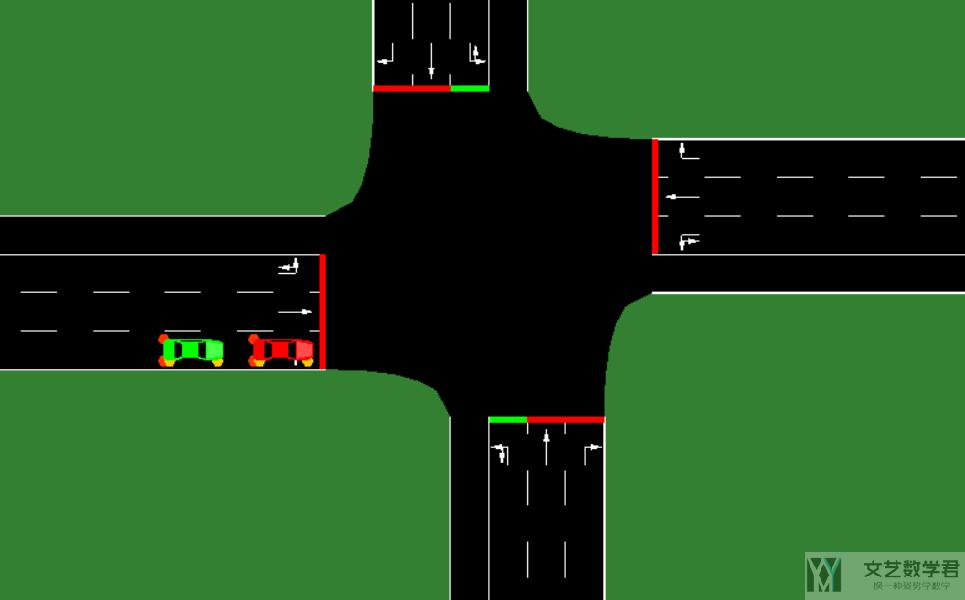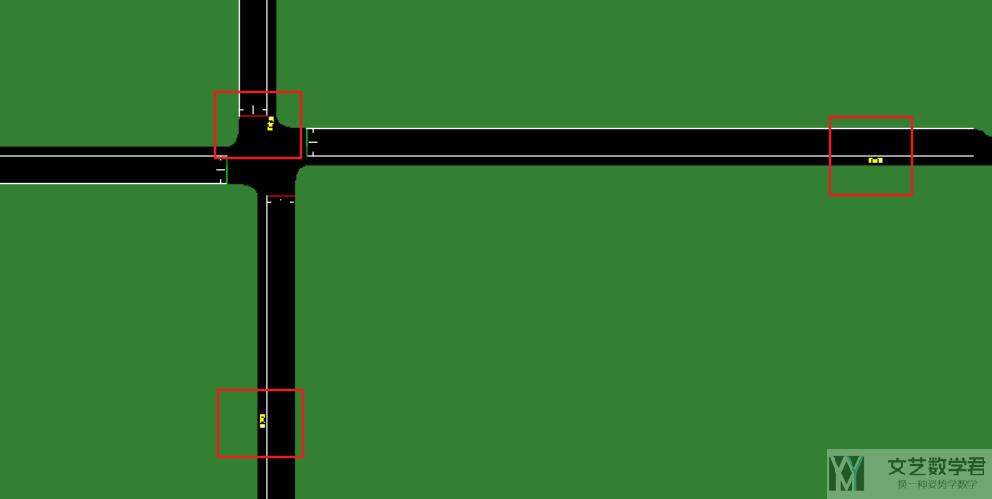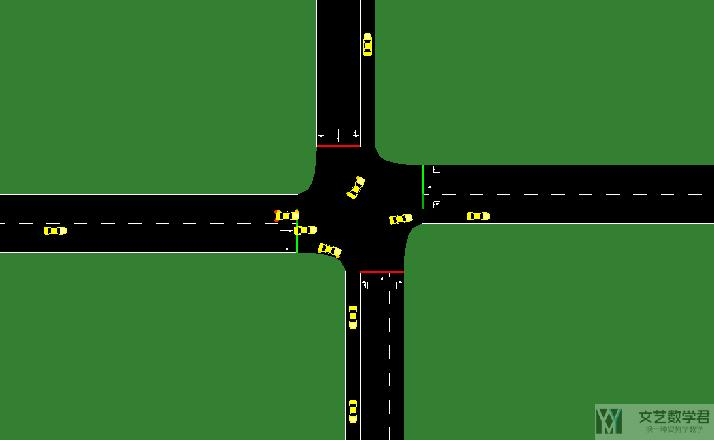# 使用 SUMO 进行仿真(3)-车流的生成（Route 文件）2021年9月25日07:25:51

12 8927字阅读29分45秒

## 生成车流（Route 文件介绍）

### 直接通过 rou.xml 文件

`SUMO` 根据 `route` 文件来生成车辆。因此最基础的生成车流的方式就是直接写 `route` 文件。这一小节会生成 `rou.xml` 文件。

1. <routes>
2.     <vType id="type1" accel="0.8" decel="4.5" sigma="0.5" length="5" maxSpeed="70"/>
3.     <route id="route0" color="1,1,0" edges="1si 3o"/>
4.     <vehicle id="0" type="type1" route="route0" depart="0" color="1,0,0"/>
5.     <vehicle id="1" type="type1" route="route0" depart="0" color="0,1,0"/>
6. </routes>
• 首先我们在最上面定义车辆，使用 `vType` 关键词进行定义。
• 接着我们定义一条路径，也就是使用 `route` 进行定义，表示车辆从哪些边上行驶。这里需要注意的是，routes 中的 edges 一定要是相互连通的，否则会出现类似下面的报错 `No connection between edge '1fi' and edge '3o'`.
• 最后我们定义了两辆车，两辆车的类型都是 type1，都在 route0 上行驶。

1. <configuration>
2.     <input>
3.         <net-file value="../hello.net.xml"/>
4.         <route-files value="hello.rou.xml"/>
5.     </input>
6. </configuration>### 通过 trip 来定义-直接指定首末位置

`.trip.xml` 文件主要保存的内容有车辆 id (id)，出发时间 (depart)，始发道路 id (from) 和到达道路 id (to)。此时我们只需要定义起点和终点，中间的路程是会自动进行补全的。我们新建一个 `hello.trip.xml` 文件，内容如下所示（一共有 3 辆车，只给定了起点和终点，没有给中间的具体道路）：

1. <routes>
2.     <trip id="trip0" depart="0" from="1fi" to="2o"/>
3.     <trip id="trip1" depart="10" from="1fi" to="3o"/>
4.     <trip id="trip2" depart="20" from="1fi" to="4o"/>
5. </routes>

1. duarouter --route-files=hello.trip.xml --net-file=../hello.net.xml --output-file=hello.rou.xml

1. <routes xmlns:xsi="http://www.w3.org/2001/XMLSchema-instance" xsi:noNamespaceSchemaLocation="http://sumo.dlr.de/xsd/routes_file.xsd">
2.     <vehicle id="trip0" depart="0.00">
3.         <route edges="1fi 1si 2o"/>
4.     </vehicle>
5.     <vehicle id="trip1" depart="10.00">
6.         <route edges="1fi 1si 3o"/>
7.     </vehicle>
8.     <vehicle id="trip2" depart="20.00">
9.         <route edges="1fi 1si 4o"/>
10.     </vehicle>
11. </routes>

1. <configuration>
2.     <input>
3.         <net-file value="../hello.net.xml"/>
4.         <route-files value="hello.rou.xml"/>
5.     </input>
6. </configuration>### 通过 flow 同时定义多个车辆（给定起点终点 duarouter）

• begin, first vehicle departure time；
• end, end of departure interval (if undefined, defaults to 24 hours)；
• vehsPerHour, number of vehicles per hour, equally spaced (not together with period or probability)；（每小时的车辆数量
• period, insert equally spaced vehicles at that period (not together with vehsPerHour or probability)；
• probability, probability for emitting a vehicle each second (not together with vehsPerHour or period), see also Simulation/Randomness；
• number, total number of vehicles, equally spaced；（给定总的车辆）

#### 通过 flow 中的 period 和 vehsPerHour 定义连续车辆

1. <routes>
2.     <!-- 定义两个车辆类型 -->
3.     <vType id="type1" accel="0.8" decel="4.5" sigma="0.5" length="5" maxSpeed="70"/>
4.     <vType id="type2" accel="1.2" decel="4.5" sigma="0.5" length="7" maxSpeed="120"/>
5.     <!-- 定义 flow, 每小时 1000 辆车 -->
6.     <flow id="flow1" color="1,1,0"  begin="0" end"7200" vehsPerHour="1000" type='type1'>
7.         <route edges="3fi 3si 4o"/>
8.     </flow>
9.     <!-- 定义 flow, 每个 5 秒有车 -->
10.     <flow id="flow2" color="0,1,1"  begin="0" end="7200" period="5" type="type2" from="1si" to="2o"/>
11. </routes>#### 通过 flow 中的 number 生成指定数量的车辆

1. <routes>
2.     <!-- 第一个时间段 -->
3.     <interval begin="0" end="100">
4.         <flow id="00" from="1si" to="2o" number="50"/>
5.     </interval>
6.     <!-- 第二个时间段 -->
7.     <interval begin="100" end="200">
8.         <flow id="10" from="1si" to="3o" number="50"/>
9.         <flow id="11" from="1si" to="4o" number="50"/>
10.     </interval>
11. </routes>

flow 文件除了上面的写法之外，还可以将 `begin``end` 写在 `flow` 里面。

1. <flow id="f2" begin="0" end="100" number="23" from="beg" to="end"/>

1. <flow id="f2" begin="0" end="100" number="23" from="beg" to="end" via="e1 e23 e7"/>

`duarouter --route-files=hello.trip.xml --net-file=../hello.net.xml --output-file=hello.rou.xml --human-readable-time --randomize-flows true --departlane random --seed 30`

• --human-readable-time，将 route 中的时间转换为 `day:hour:minute:second` 的格式，而不是只有 second；
• --randomize-flows，将车辆 depart time 随机，而不是均匀出现；
• --departlane，车辆起始的车道，我们选择 random；
• --seed，随机数种子，用来控制多次运行最终的结果是相同的；

1. <routes xmlns:xsi="http://www.w3.org/2001/XMLSchema-instance" xsi:noNamespaceSchemaLocation="http://sumo.dlr.de/xsd/routes_file.xsd">
2.     <vehicle id="00.0" depart="00:00:070.58" departLane="random">
3.         <route edges="1si 2o"/>
4.     </vehicle>
5.     <vehicle id="00.1" depart="00:00:070.91" departLane="random">
6.         <route edges="1si 2o"/>
7.     </vehicle>
8. ...
9. </routes>

#### 使用 flow 定义时定义车辆类型

1. <routes>
2.     <!-- 定义车辆类型 -->
3.     <vType id="type1" accel="2.6" decel="4.5" sigma="0.5" length="5" maxSpeed="70"/>
4.     <vType id="type2" accel="2.6" decel="4.5" sigma="0.5" length="5" maxSpeed="70"/>
5.     <!-- 定义车流 -->
6.     <flow id="221888670#10__01" begin="0" end="60" from="221888670#10" number="8" type="type1"/>
7.     <flow id="221888670#10__02" begin="0" end="60" from="221888670#10" number="8" type="type2"/>
8. <routes>

### 通过 TAZ（traffic assignment zone）来进行定义

2.    <taz id="TAZ_1" edges="1fi 4si"/>
3.    <taz id="TAZ_2" edges="2si 3si"/>

1. <routes>
2.     <interval begin="0" end="100">
3.         <flow id="1" depart="0" fromTaz="TAZ_1" toTaz="TAZ_2" number="50"/>
4.     </interval>
5. </routes>

1. duarouter --route-files=cross.trip.xml --net-file=cross.net.xml --taz-files=cross.taz.xml --output-file=cross.rou.xml

### 通过 randomTrips.py 生成车流

1. python randomTrips.py -n input_net.net.xml

1. python randomTrips.py --help

### 通过 Turn Probabilities 来生成车辆（jtrrouter）

1. <edgeRelations>
2.    <interval begin="0" end="3600">
3.       <edgeRelation from="1si" to="3o" probability="0.4"/>
4.       <edgeRelation from="1si" to="2o" probability="0.4"/>
5.       <edgeRelation from="1si" to="4o" probability="0.2"/>
6.    </interval>
7. </edgeRelations>

1. python "C:\Program Files (x86)\Eclipse\Sumo\tools\turn-defs\generateTurnDefs.py" --connections-file wj.net.xml --turn-definitions-file testflow.turndefs.xml

1. no declaration found for element 'edgeRelation'

1. xmlns:xsi="http://www.w3.org/2001/XMLSchema-instance" xsi:noNamespaceSchemaLocation="http://sumo.dlr.de/xsd/turns_file.xsd"

1. <routes>
2.     <flow id="0" from="1si" begin="0" end="3600" number="1000"/>
3. </routes>

1. python "randomTrips.py" --net-file=wj.net.xml --flows 3 --jtrrouter

1. jtrrouter --route-files=hello.trip.xml --turn-ratio-files=hello.turndefs.xml --net-file=../hello.net.xml --accept-all-destinations true --output-file=hello.rou.xml• --allow-loops <BOOL>, Allow to re-use a road; default: false
• --remove-loops <BOOL> Remove loops within the route; Remove turnarounds at start and end of the route; default: false

• 微信公众号
• 关注微信公众号
•• QQ群
• 我们的QQ群号
•• 本文由 发表于 2021年9月25日07:25:51
• 转载请务必保留本文链接：https://mathpretty.com/14077.html